## FCN

• 全卷积化（Convolutionalization）：跟之前差不多，但是去掉了全连接层
• 反卷积（Deconvolution）：用在把小的feature map上采样上去
• 跳层结构（Skip Layer）：就是把不同层柔和到一起的方法

### 反卷积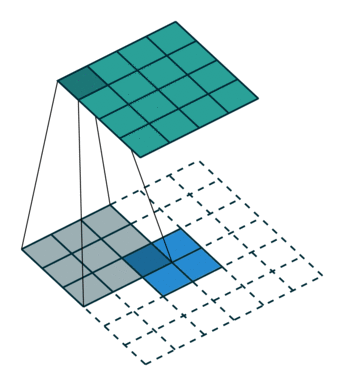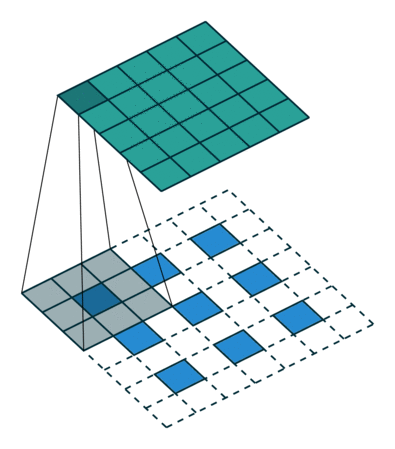### 跳层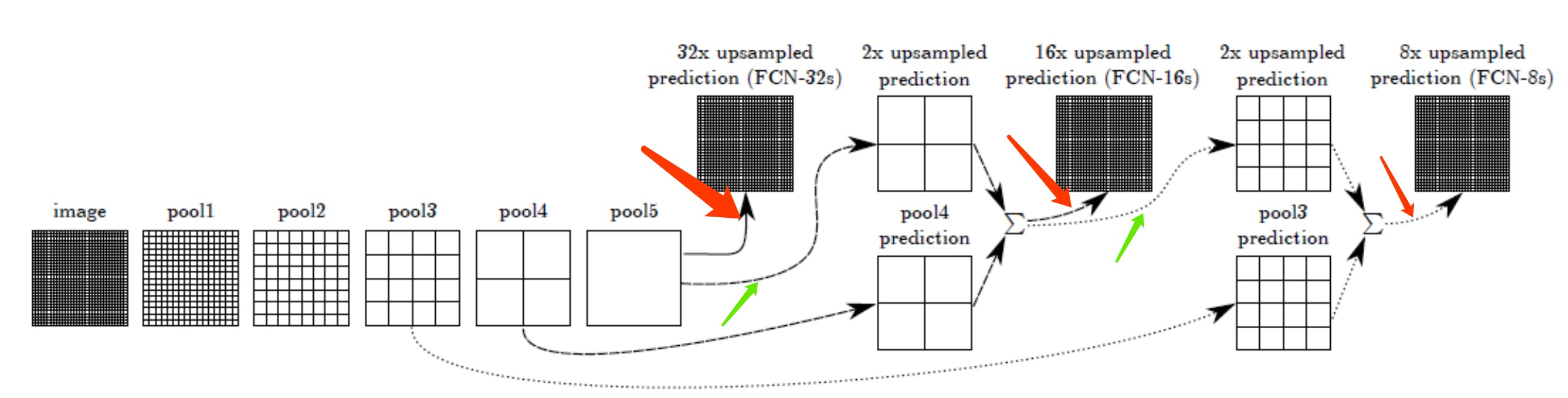• FCN-16s就是pool4+pool5的信息，柔和到一起
• FCN-8s就是pool3+pool4+pool5的信息，柔和到了一起，是最精细的了。

### ResNET50的FCN

FCN的backbone可以是任何主流的backbone，常用的ResNet50应该是怎么样的呢？我们来详细说说。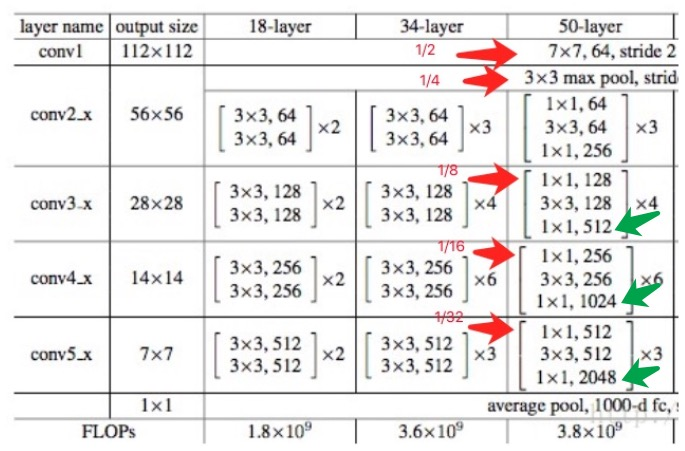#conv1 ->1/2 , 1/4
x = Conv2d_BN(x, nb_filter=64, kernel_size=(7, 7), strides=(2, 2), padding='valid')
x = MaxPooling2D(pool_size=(3, 3), strides=(2, 2), padding='same')(x)

#conv3_x ->1/8
x = identity_Block(x, nb_filter=128, kernel_size=(3, 3), strides=(2, 2), with_conv_shortcut=True)

#conv4_x ->1/16
x = identity_Block(x, nb_filter=256, kernel_size=(3, 3), strides=(2, 2), with_conv_shortcut=True)

#conv5_x ->1/32
x = identity_Block(x, nb_filter=512, kernel_size=(3, 3), strides=(2, 2), with_conv_shortcut=True)


Identity_Block,是Resnet设计的一个可重复的卷基层，由3组个卷积、BatchNormal、Relu激活组成，就是上图中中括弧表示的内容。

• 1/2的时候：line 143:x = Conv2D(64, (7, 7), data_format=IMAGE_ORDERING,strides=(2, 2), name='conv1')(x)
• 1/4的时候：line 149:x = MaxPooling2D((3, 3), data_format=IMAGE_ORDERING, strides=(2, 2))(x)
• 1/8的时候：line 151:x = conv_block(x, 3, [128, 128, 512], stage=3, block='a')
• 1/16的时候：line 162:x = conv_block(x, 3, [256, 256, 1024], stage=4, block='a')
• 1/32的时候：line 170:x = conv_block(x, 3, [512, 512, 2048], stage=5, block='a')

### 反卷积的实现

TF的函数tf.nn.conv2d_transpose

• value的格式为[batch, height, width, in_channels]，height和width是用来计算输出尺寸用到的最重要的两个参数，表示输入该层feature map的高度和宽度，典型的NHWC格式；
• filter的格式为[height, width, output_channels, input_channels]，务必注意这里的channel数是输出的channel数在前，输入的channel数在后。
• output_shape是一个1-D张量，传入的可以是一个tuple或者list，在不指定data_format参数的情况下，格式必须为NHWC。注意：这里的C要与filter中的output_channels保持一致；
• strides的格式为一个整数列表，与conv2d方法在官方文档中写的一样，必须保证strides=strides=1，格式为[1, stirde, stride, 1]；
• （与conv2d方法不同的是，这里需要人为指定输出的尺寸，这是为了使用value、output_shape和strides三个参数一起确定反卷积尺寸的正确性，具体在下个部分解释。）

### FCN的实现

• Keras实现
  o = f5
o = (Conv2D(4096, (7, 7), activation='relu',padding='same', data_format=IMAGE_ORDERING))(o)
o = Dropout(0.5)(o)
o = (Conv2D(4096, (1, 1), activation='relu',padding='same', data_format=IMAGE_ORDERING))(o)
o = Dropout(0.5)(o)
o = (Conv2D(n_classes,  (1, 1), kernel_initializer='he_normal',data_format=IMAGE_ORDERING))(o)
o = Conv2DTranspose(n_classes, kernel_size=(64, 64),  strides=(32, 32), use_bias=False,  data_format=IMAGE_ORDERING)(o)


看！反卷积之前，对原始的feature map做了3次卷积（7x7和1x1和1x1）

• Tensorflow实现
  conv_final_layer = image_net["conv5_3"]
pool5 = utils.max_pool_2x2(conv_final_layer)
W6 = utils.weight_variable([7, 7, 512, 4096], name="W6")
conv6 = utils.conv2d_basic(pool5, W6, b6)<--------------7x7卷积
relu6 = tf.nn.relu(conv6, name="relu6")
W7 = utils.weight_variable([1, 1, 4096, 4096], name="W7")
conv7 = utils.conv2d_basic(relu6, W7, b7)<---------------1x1卷积
relu7 = tf.nn.relu(conv7, name="relu7")
W8 = utils.weight_variable([1, 1, 4096, NUM_OF_CLASSESS], name="W8")
conv8 = utils.conv2d_basic(relu7, W8, b8)<---------------1x1卷积
W_t1 = utils.weight_variable([4, 4, deconv_shape1.value, NUM_OF_CLASSESS], name="W_t1")
conv_t1 = utils.conv2d_transpose_strided(conv8, W_t1, b_t1, output_shape=tf.shape(image_net["pool4"]))<----------------反卷积（这个是pool5经过一些列卷加后的反卷积，strip=2）


这块有个疑问？pool5经过卷积后的conv8，为何要上卷积呢？论文里明明是2 x unsampled，也就是反池化啊？！

不过，这里还是跟上面的做法一样，反卷积之前，对原始的feature map做了3次卷积（7x7和1x1和1x1）。

• Caffi的实现,
  n.fc6, n.relu6 = conv_relu(n.pool5, 4096, ks=7, pad=0)
n.fc7, n.relu7 = conv_relu(n.drop6, 4096, ks=1, pad=0)
n.score_fr = L.Convolution(n.drop7, num_output=60, kernel_size=1, pad=0,param=[dict(lr_mult=1, decay_mult=1), dict(lr_mult=2, decay_mult=0)])
n.upscore = L.Deconvolution(n.score_fr,convolution_param=dict(num_output=60, kernel_size=64, stride=32,bias_term=False)


也是7x7卷积，2个1x1卷积后，才做反卷积

• Pytorch实现
      self.pool5 = nn.MaxPool2d(2, stride=2, ceil_mode=True)  # 1/32
self.fc6 = nn.Conv2d(512, 4096, 7)<----7x7卷积
self.relu6 = nn.ReLU(inplace=True)
self.fc7 = nn.Conv2d(4
。
pool5是只做了一个crop剪切，, 1)<----1x1卷积
self.relu7 = nn.ReLU(inplace=True)
self.score_fr = nn.Conv2d(4096, n_class, 1)<----1x1卷积
self.upscore = nn.ConvTranspose2d(n_class, n_class, 64, stride=32,bias=False)


一样一样，都是7x7,1x1,1x1三个卷积。

## UNet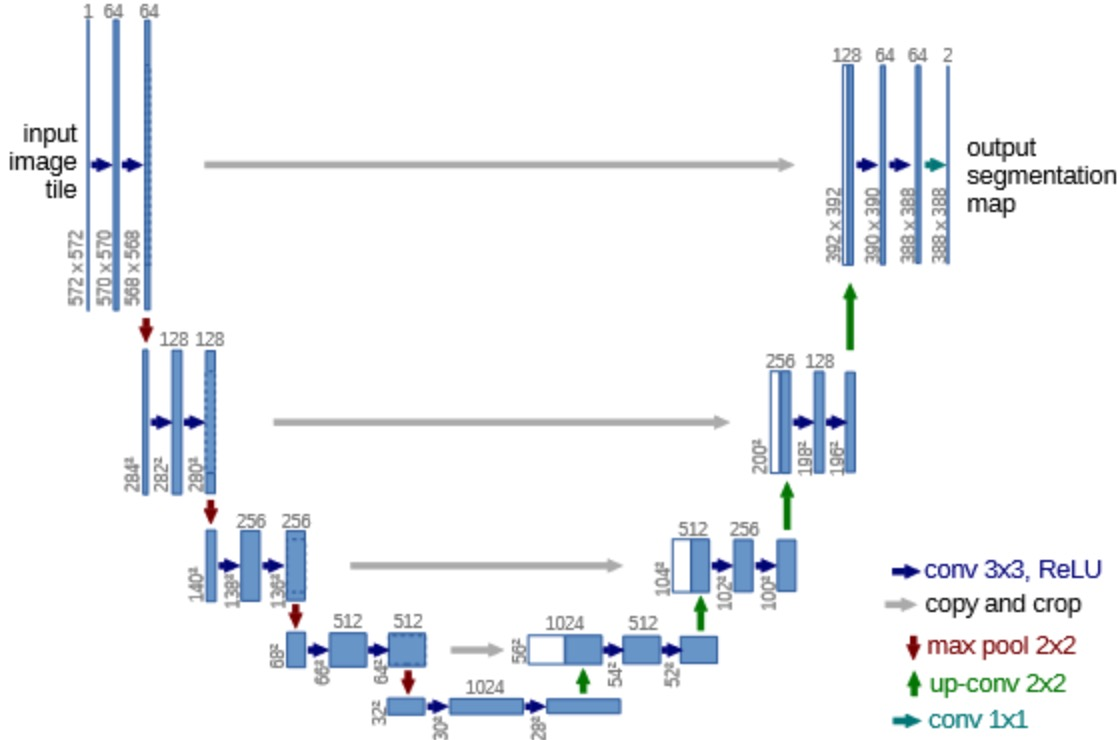### UNet和FCN的区别

Unet实际上是改进了FCN，究竟做了哪些改进呢？

### 大体思路

1，输入一幅你想处理的图片，然后进行对应的预处理操作，或者预处理后的图片；
2，将其输入到一个预训练好的神经网络中（ResNeXt等）获得对应的feature map；
3，对这个feature map中的每一点设定预定个的ROI，从而获得多个候选ROI；
5，对这些剩下的ROI进行ROIAlign操作（即先将原图和feature map的pixel对应起来，然后将feature map和固定的feature对应起来）（ROIAlign为本文创新点1，比ROIPooling有长足进步）；


#### Fast-RCNN

RCNN还是非常慢，大约会搞出2000个selective search得到的ROI，然后每个ROI都要过卷积CNN进行计算，慢死了！#### Faster-RCNN#### FPN

FPN和FCN名字很像，样子又很像UNet，特容易混。恩，其实，在我看来FPN就是借鉴了FCN，用同样的办法，融合了不同感受野的feature map。

【FCN、UNet、FPN的关系和区别】

FCN是鼻祖，2014.11年就出来了，2015年3最终稿，它是开山之作。为了解决XXX问题。

UNet是2015.5发表，FPN都是2016.12发表，终稿是2017.3的。他俩都是站在FCN的基础之上。

• FPN出自detection任务；U-Net出自segmentation任务
• FPN的“放大”部分是直接插值放大的，没有deconvolution的filters学习参数；U-Net“放大”部分就是Decoder，需要deconvolution的filters学习参数的。
• FPN及其大多数改进都是把原Feature Map和FPN的Feature Map做加法；U-Net及其大多数改进都是把原Feature Map和Decoder的Feature Map做Concatiantion，再做1x1卷积。
• FPN对每一个融合的层都做detection；U-Net 只在最后一层做segmentation的pixel预测。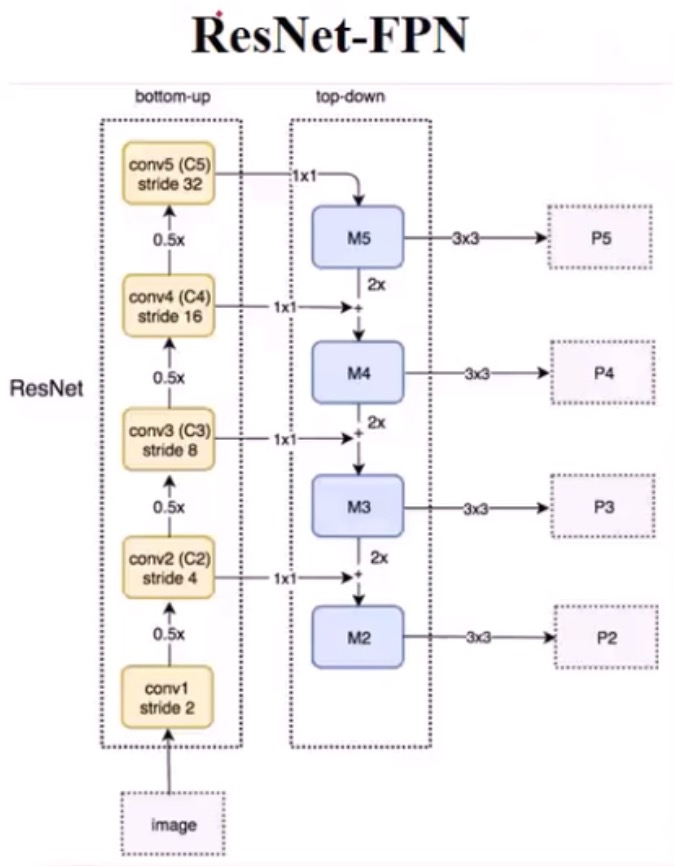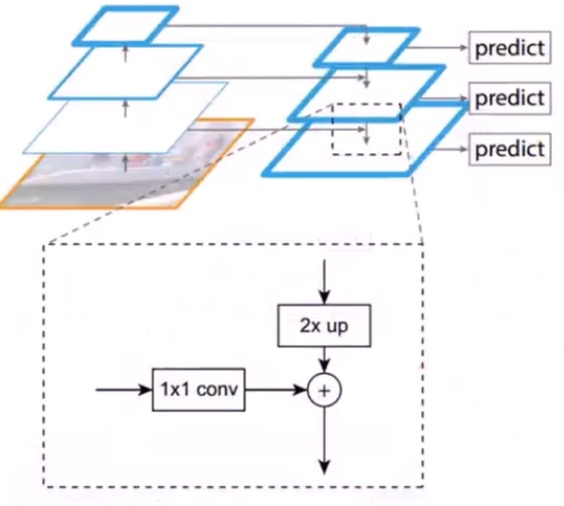FPN还搞了一个P6，就是resetnet的c5，再做一次下采样的结果，干嘛用呢，用来做RPN的输入，就是产生那些anchor们用的。而p5,p4,p3,p2，都用于产生每个anchor的回归和置信度的结果用了。可以产生，恩，4个批，4个不同层次的。

FPN+RPN+Fast-RCNN⇒牛逼的faster-rcnn，比之前的faster-rcnn牛逼的地方就在于多尺度了！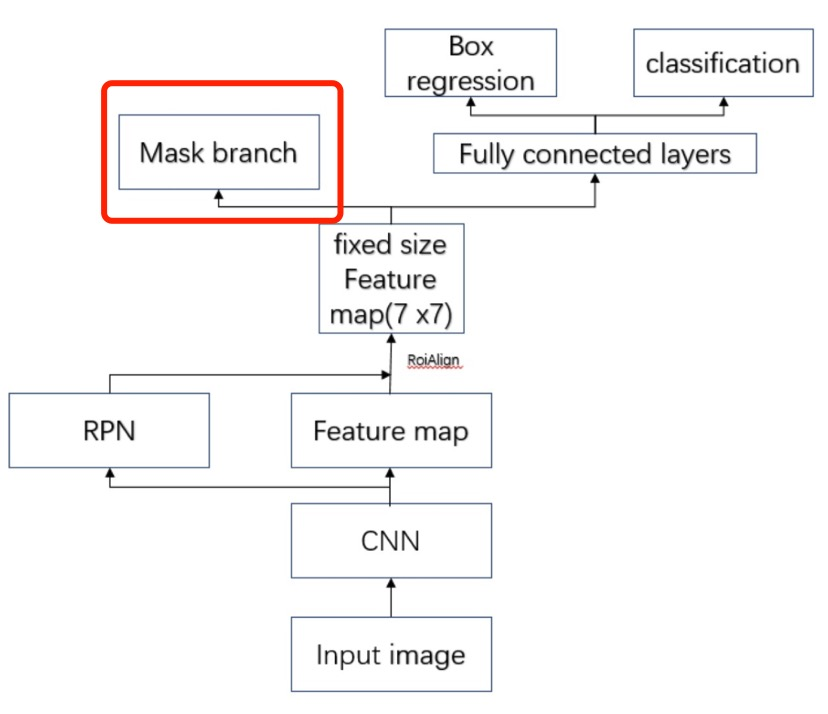• 创新点1：改进了ROI Align，使用双线性插值，比ROIPooling有长足进步
• 创新点3：损失函数的计算，作者放弃了更广泛的softmax，转而使用了sigmoid，避免了同类竞争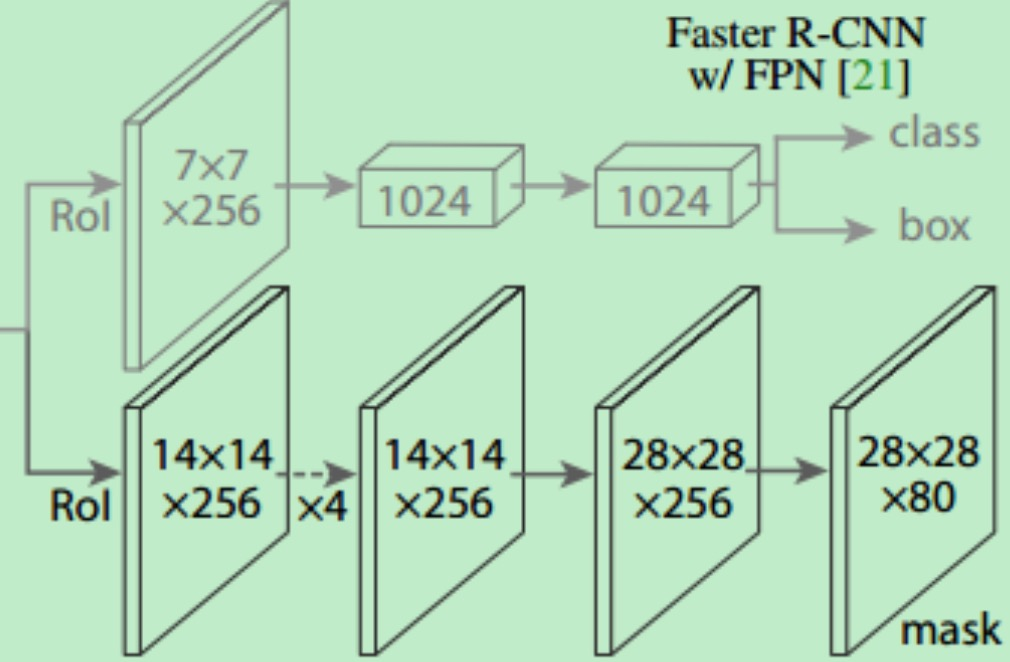#### 双线性插值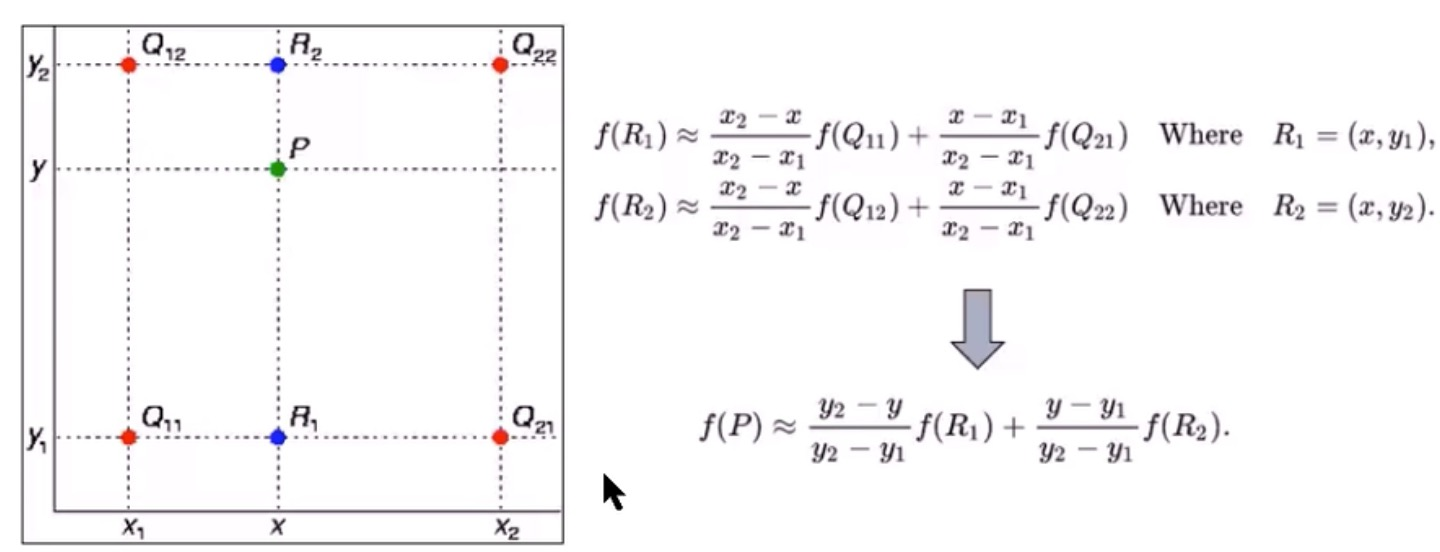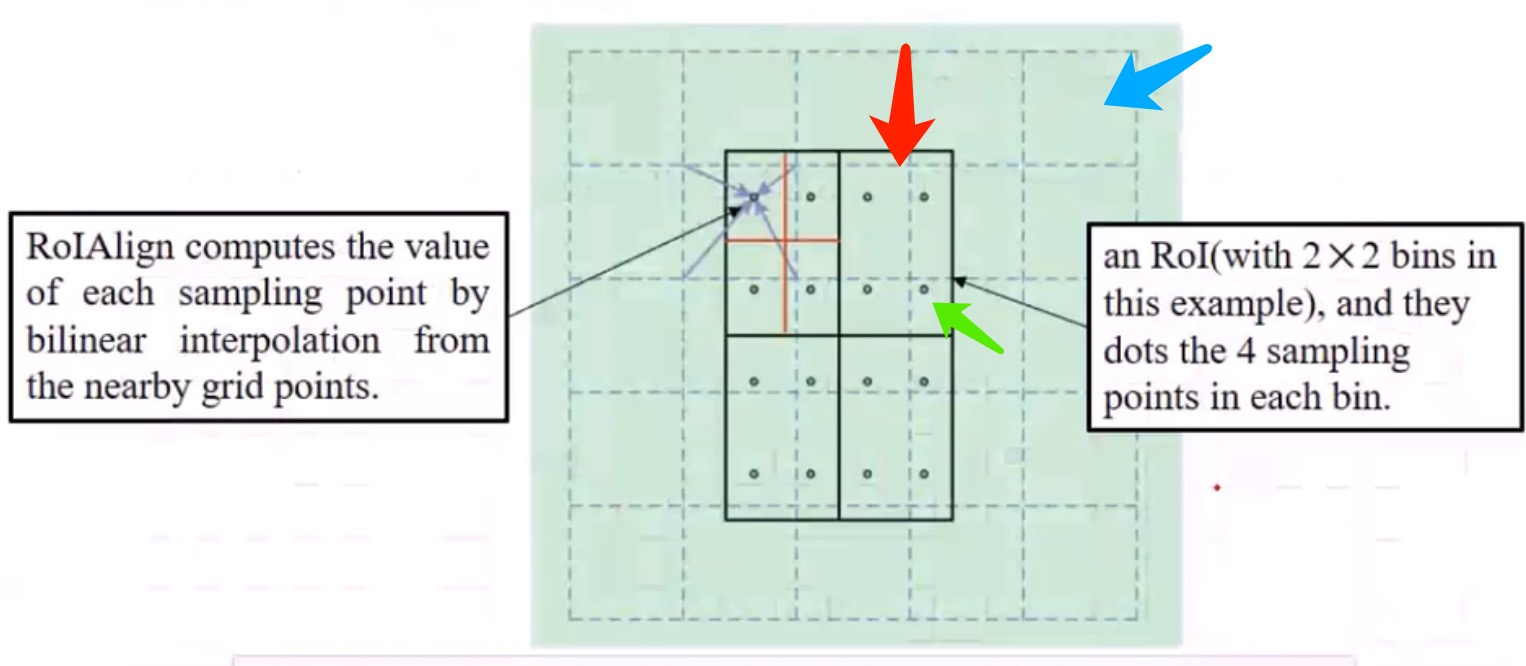【视频】

【文章】